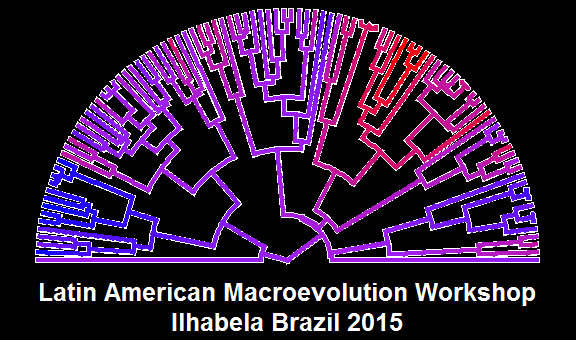## Friday, June 12, 2015

### T-shirt design for Ilhabela workshop - in R

I'm in the midst of working on a design for the t-shirts for this year's macroevolution workshop in Ilhabela, Brazil. I've created a tentative 'front' design entirely in R using the function of phytools, as follows:

``````library(phytools)
## don't run
# tree<-pbtree(n=100)
# x<-fastBM(tree)
``````
``````obj<-contMap(tree,x,plot=FALSE)
obj<-setMap(obj,colors=c("blue","purple","red"))
layout(mat=matrix(c(1,2),2,1),heights=c(0.8,0.2))
par(bg="black")
par(fg="white")
plotSimmap(paintSubTree(tree,Ntip(tree)+1,"1"),type="fan",
ftype="off",colors=setNames("white","1"),lwd=6,part=0.5)
``````
``````## setEnv=TRUE for this type is experimental. please be patient with bugs
``````
``````plotSimmap(obj\$tree,type="fan",ftype="off",colors=obj\$cols,lwd=4,
``````
``````## setEnv=TRUE for this type is experimental. please be patient with bugs
``````
``````plot.new()
text(0.5,0.5,"Latin American Macroevolution Workshop\nIlhabela Brazil 2015",
col="white",cex=2.1,font=2)
``````The knitr output is kind of aliased. We can create a higher quality PDF easily:

``````pdf(file="t-shirt.pdf",width=8,height=4.75)
layout(mat=matrix(c(1,2),2,1),heights=c(0.8,0.2))
par(bg="black")
par(fg="white")
plotSimmap(paintSubTree(tree,Ntip(tree)+1,"1"),type="fan",
ftype="off",colors=setNames("white","1"),lwd=6,part=0.5)
``````
``````## setEnv=TRUE for this type is experimental. please be patient with bugs
``````
``````plotSimmap(obj\$tree,type="fan",ftype="off",colors=obj\$cols,lwd=4,
``````
``````## setEnv=TRUE for this type is experimental. please be patient with bugs
``````
``````plot.new()
text(0.5,0.5,"Latin American Macroevolution Workshop\nIlhabela Brazil 2015",
col="white",cex=2.1,font=2)
dev.off()
``````
``````## windows
##       2
``````

Here is a link to the file. (It looks much better!)

That's it for now.